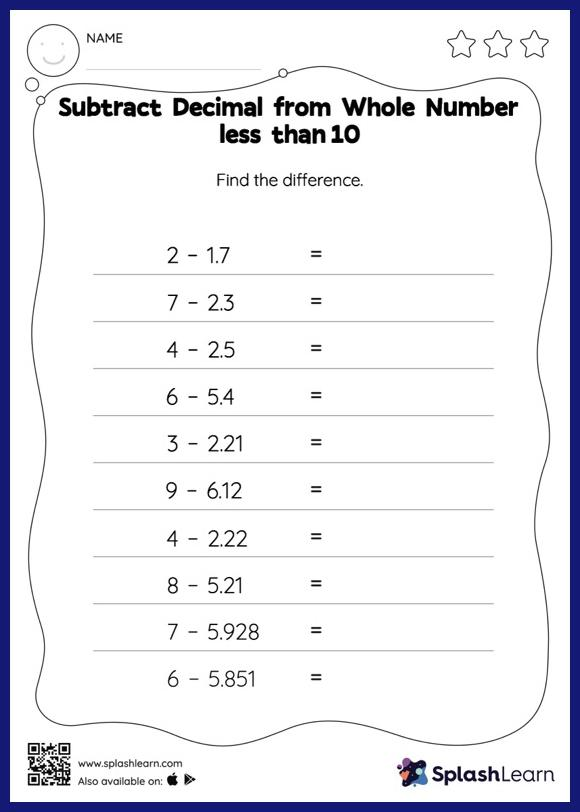# Subtract Decimal from Whole Number less than 10: Horizontal Subtraction Worksheet

Home > Subtract Decimal from Whole Number less than 10: Horizontal SubtractionEvery 5th graders needs this worksheet to master decimals. Aid your child on their learning journey as they practice with subtract decimals.